# 2-Way Directional Valve (TL)

2-way directional valve in a thermal liquid network

•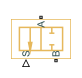Libraries:
Simscape / Fluids / Thermal Liquid / Valves & Orifices / Directional Control Valves

## Description

The 2-Way Directional Valve (TL) block represents a two-way valve, such as a shut-off valve. Use this block to simulate a flow-reducing control element that responds to pressures in another part of the system.

The block uses a variable orifice to control the flow between ports A and B. The physical signal at port S triggers the spool motion to open or shut the valve. For more details about how the block calculates flow rate through a variable orifice, see Orifice (TL).

### Orifice Parameterizations

You can parameterize the valve opening linearly or by using tabulated data.

Linear

When you set Orifice parameterization to `Linear - Area vs. spool travel`, the opening area is a linear function of the spool travel distance.

`${A}_{orifice}=\frac{\left({A}_{\mathrm{max}}-{A}_{leak}\right)}{\Delta {S}_{\mathrm{max}}}\Delta S+{A}_{leak},$`

where

• Aorifice is the opening area.

• ΔS is the spool travel distance input at port S. $\Delta S=S-{S}_{\mathrm{max}}+\Delta {S}_{\mathrm{max}}.$

• ΔSmax is the value of the Spool travel between closed and open orifice parameter.

• ALeak is the value of the Leakage area parameter.

• Amax is the value of the Maximum orifice area parameter.

Note the linear scaling from ALeak to Amax in the figure.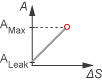When the valve is in a near-open or near-closed position in the linear parameterization, you can maintain numerical robustness in your simulation by adjusting the parameter. If the parameter is nonzero, the block smoothly saturates the opening area between Aleak and Amax. For more information, see Numerical Smoothing.

Tabulated

When you set Orifice parameterization to `Tabulated data - Area vs. spool travel`, the block uses the Spool travel vector and Orifice area vector parameters to define the relationship between Aorifice and ΔS by interpolation. Aleak and Amax are the first and last parameters of the Orifice area vector parameter, respectively.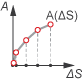When you set Orifice parameterization to ```Tabulated data - Volumetric flow rate vs. spool travel and pressure drop```, the block uses the Volumetric flow rate table, q(ds,dp), Pressure drop vector, dp, and Spool travel vector, ds parameters to define the volumetric flow rate, q(ΔS,p).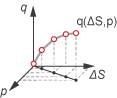When you set Orifice parameterization to ```Tabulated data - Mass flow rate vs. opening and pressure drop``` the block calculates the mass flow rate directly from the spool position and the pressure drop across the valve. The relationship between the three variables can be nonlinear and it is given by the tabulated data in the Spool travel vector, ds, Pressure drop vector, dp, and Mass flow rate table, mdot(ds,dp) parameters.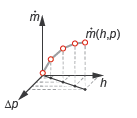### Visualize Orifice Openings

To visualize the spool offsets and displacement, right-click the block and select Fluids > Plot Valve Characteristics. The plot shows the valve open area or volumetric flow rate as a function of spool position.

To update the data after changing the block parameters, click in the figure window.

This figure shows the valve configuration with:

• Spool position at maximum orifice area set to `2e-3` m.

• Spool travel between closed and open orifice set to `6e-3` m.

All other parameters are at the default values.## Ports

### Conserving

expand all

Thermal liquid conserving port.

Thermal liquid conserving port.

### Input

expand all

Physical signal associated with the spool displacement, in m. A positive value causes the valve to open.

## Parameters

expand all

### Model Parameterization

Method the block uses to calculate the valve flow area.

Position of the spool travel member when the valve is fully open.

Spool offset at the maximum valve opened area.

#### Dependencies

To enable this parameter, set Orifice parametrization to ```Linear - area vs. spool travel```.

Maximum valve area experienced during simulation.

#### Dependencies

To enable this parameter, set Orifice parametrization to ```Linear - area vs. spool travel```.

Sum of all gaps when the valve is in the fully closed position. The block maintains any area smaller than this value at the specified leakage area. This parameter contributes to numerical stability by maintaining continuity in the flow.

#### Dependencies

To enable this parameter, set Orifice parametrization to ```Linear - area vs. spool travel```.

Continuous smoothing factor that introduces a layer of gradual change based to the flow response when the valve is in near-open and near-closed positions. To increase the stability of your simulation in these regimes, set this parameter to a nonzero value less than one.

#### Dependencies

To enable this parameter, set Orifice parametrization to ```Linear - area vs. spool travel```.

Vector of control member travel distances. A positive value opens the valve. The values in this vector correspond one-to-one to values in the Orifice area vector parameter. The values must ascend from 0. The block interpolates the points linearly.

#### Dependencies

To enable this parameter, set Orifice parametrization to ```Tabulated data - Area vs. spool travel```.

Vector of valve area values for the tabular parametrization of opening area. The values in this vector correspond one-to-one with the elements in the Spool travel vector parameter. The first element of this vector is the leakage area, and the last element is the maximum valve area. The block interpolates the points linearly.

#### Dependencies

To enable this parameter, set Orifice parametrization to ```Tabulated data - Area vs. spool travel```.

Discharge loss for a hydraulic structure.

#### Dependencies

To enable this parameter, set Orifice parametrization to either:

• ```Tabulated data - Area vs. spool travel```

• ```Linear - area vs. spool travel```

Upper Reynolds number limit for laminar flow through the valve.

#### Dependencies

To enable this parameter, set Orifice parametrization to either:

• ```Tabulated data - Area vs. spool travel```

• ```Linear - area vs. spool travel```

Whether to account for pressure increase when the fluid flows from a region of smaller cross-sectional area to a region of larger cross-sectional area.

Vector of control member travel distances. The spool travel vector forms an independent axis with the Pressure drop vector, dp parameter for the 3-D dependent Volumetric flow rate table, q(ds,dp) parameter. A positive displacement corresponds to valve opening. The values ascend from 0. The block interpolates the points linearly.

#### Dependencies

To enable this parameter, set Orifice parametrization to ```Tabulated data - Volumetric flow rate vs. spool travel and pressure drop```.

Vector of pressure drop values for the volumetric flow rate. The pressure drop vector forms an independent axis with the Spool travel vector, ds parameter for the 3-D dependent Volumetric flow rate table, q(ds,dp) parameter. The values must be ascending. The block interpolates the points linearly

#### Dependencies

To enable this parameter, set Orifice parametrization to ```Tabulated data -Volumetric flow rate vs. spool travel and pressure drop```.

M-by-N matrix of volumetric flow rates based on independent values of pressure drop and spool travel distance. M and N are the sizes of the correlated vectors:

• M is the number of elements in the parameter.

• N is the number of elements in the Pressure drop vector, dp parameter.

#### Dependencies

To enable this parameter, set Orifice parametrization to ```Tabulated data - Volumetric flow rate vs. spool travel and pressure drop```.

M-by-N matrix of volumetric flow rates based on independent values of pressure drop and spool travel distance. M and N are the sizes of the correlated vectors:

• M is the number of elements in the parameter.

• N is the number of elements in the Pressure drop vector, dp parameter.

#### Dependencies

To enable this parameter, set Orifice parametrization to ```Tabulated data - Mass flow rate vs. spool travel and pressure drop```.

Temperature at which the tabulated mass flow rate data is specified.

#### Dependencies

To enable this parameter, set Orifice parameterization to ```Tabulated data - Mass flow rate vs. spool travel and pressure drop```.

Pressure at which the tabulated mass flow rate data is specified. The block uses this parameter to calculate a third reference parameter, the reference density.

#### Dependencies

To enable this parameter, set Orifice parameterization to ```Tabulated data - Mass flow rate vs. spool travel and pressure drop```.

Cross-sectional area at ports A and B. The block uses this area in the pressure-flow rate equation to determine the volumetric flow rate through the valve.

#### Dependencies

To enable this parameter, set Orifice parametrization to either:

• ```Tabulated data - Area vs. spool travel```

• ```Linear - area vs. spool travel```

## Version History

Introduced in R2016a

expand all